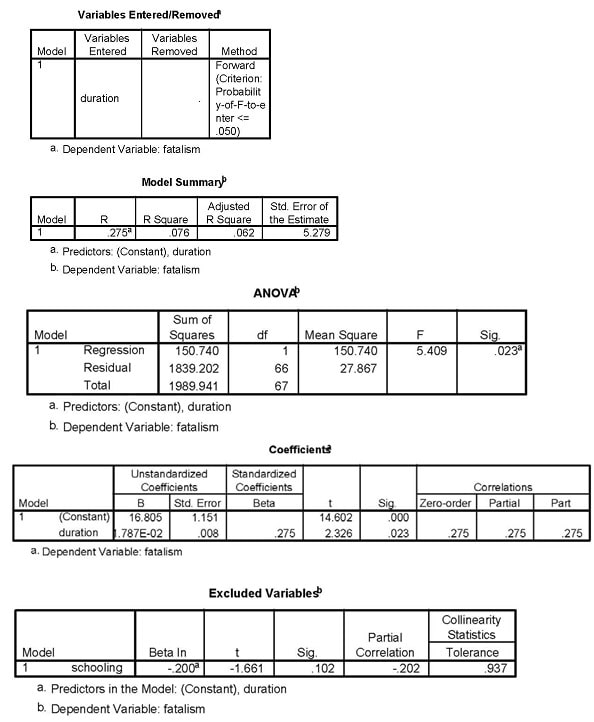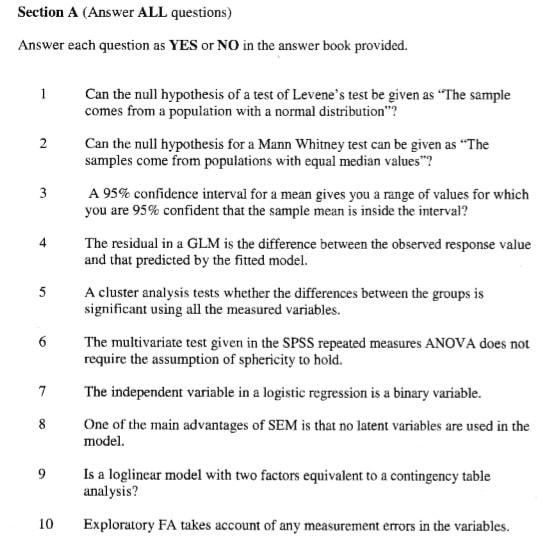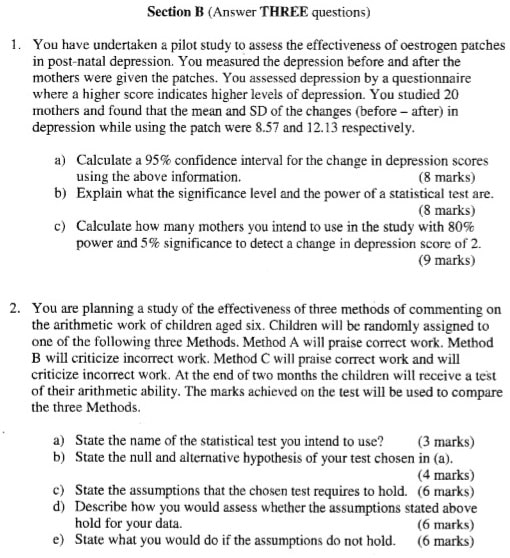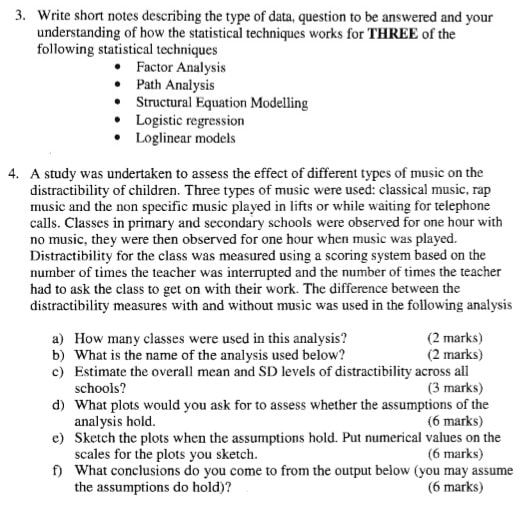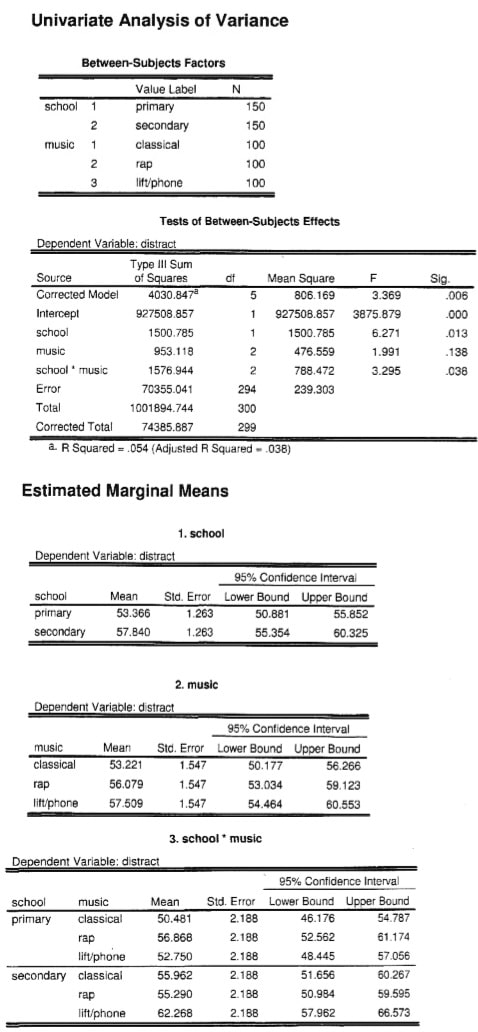```PHP4006
Bangor University
```
1. You are planning a study of the effectiveness of two therapies for treating Depression. Group A will receive the current therapy and Group B will receive a new and improved therapy. Participants are randomly assigned to one of the therapy groups. A measure of depression is taken twice: before and after therapy. You are trying to decide if the new therapy is more effective than the current approach.
1. State the name and design of the statistical test you intend to use? (3 marks)
2. Justify the use of the chosen test in (a). (6 marks)
3. State the null hypotheses of your test chosen in (a). (3 marks)
4. Explain what the statistical power of the chosen test is. (4 marks)
5. State the assumptions that the chosen test requires to hold. (4 marks)
6. State one other statistical test you would consider using in this situation and explain its design. (5 marks)
2. Although it is well-established that there is a strong biological factor underlying Anorexia Nervosa, you are interested in psychological factors that relate to this condition. You have collected data from adolescent girls assessed at an Eating Disorder Clinic along with an age-matched control sample. The data includes the following: whether or not the girls were diagnosed with Anorexia; whether or not there is a family history of depression; and a questionnaire that measures feelings of Ineffectiveness. While the Ineffectiveness scale potentially ranges from 0-50, you are undecided whether to use a median split to define two discrete groups (i.e., High vs. Low Ineffectiveness).
1. Determine the form of the ineffectiveness variable, and state the name of the statistical test you intend to use. (3 marks)
2. Justify the use of the chosen test in (a). (6 marks)
3. Explain the basic inferences which can be derived from this data using your chosen test. (8 marks)
4. State the assumptions that the chosen test requires to hold. (5 marks)
5. State the name statistical test you would intend to use for the alternative form of the Ineffectiveness variable. (3 marks)
3. You have studied the impact of physiological arousal on problem-solving ability. You measured problem-solving using a task where a higher score represents better problem-solving. 60 participants were allocated to one of three conditions: a control condition and two conditions inducing physiological arousal (Mild or High). After the treatment, participants completed the problem-solving task. The output for the One-way independent ANOVA is below (assumptions were met).
1. Calculate the Eta-squared effect size for the differences in problemsolving across conditions from the information below. (3 marks)
2. Calculate the Pearson’s r effect size for ONE contrast using the information below. (5 marks)
3. Explain some advantages of using effect sizes in addition to measures of statistical significance. (4 marks)
4. Briefly summarise the main findings of the data below. (6 marks)
5. Explain why a trend analysis would be informative for this design and, given the data, what would you expect it to show? (5 marks)
6. If the assumptions were not met for ANOVA, which statistical test would you consider using to examine these data? (3 marks)
```                                           Descriptives
Problem Solving

95% Confidence Interval for
Std. 	                                 Mean

N      Mean        Deviation  Std. Error  Lower Bound Upper Bound   Min 	Max
Control     20 	   84.05       10.31 	  2.31 	      79.22 	  88.88 	63.00 	101.00
Mid 	    20 	   96.80       12.12 	  2.71 	      91.13 	 102.47 	72.00 	114.00
High 	    20 	   88.00       11.84 	  2.65 	      82.46 	  93.54 	65.00 	102.00
Total 	    60 	   89.62       12.47 	  1.61 	      86.39 	   92.84 	63.00 	114.00
```
 Problem Solving ANOVA Sum of Squares df Mean Square F Sig. Between Groups 1704.033 2 852.017 6.496 .003 Within Groups 7476.150 57 131.161 Total 9180.183 59

Contrast Coefficients

 Contrast Control Arousal Condition Mid High 1 -2 1 1 2 0 -1 1

Contrast Tests

 Contrast Value of Contrast Std. Error t df Sig. (2-tailed) Problem Solving Assume equal 1 variances 2 16.70 -8.80 6.27 3.62 2.662 -2.430 57 57 .010 .018 Does not assume 1 equal variances 2 16.70 -8.80 5.97 3.79 2.798 -2.323 43.41 37.98 .008 .026
1. The data on the following pages are on glucose control in diabetic patients.

Good control is measured by a low value of Glucose (the outcome or Y value).

 outcome G Glucose in the blood predictors K Knowledge of the illness F Measure of attribution called fatalistic externalism D Duration of the illness in months S Length of schooling 0 – less than 13 years, 1 – more than 13 years

The variables above were measured on diabetic patients. A theory proposes that glucose is directly affected by knowledge and duration, knowledge is directly affected by fatalism, and fatalism is directly affected by duration. The theory does not propose any effect of schooling.

The output below is taken from the use of Forward regressions to predict each of glucose, knowledge, and fatalism from the variables suggested by the above theory. Schooling was included as a possible predictor in each regression.

• Draw the path diagram that the above model proposes.
• Comment on the correlation coefficients between schooling and the other 4 variables.
• Explain the difference between the Forward and Backward methods of regression.
• Using the determined model in Regression 1 what equation would you use to predict glucose, and predict the Glucose level of a person with Knowledge = 36,

Duration = 143, Schooling = 0?

• Use the regression outputs to enter the path coefficients for the original theoretical model (write them in the path diagram you drew earlier){`>`}marks)
• Use the data below to specify a second model which better accounts for all the data, and enter the appropriate path coefficients. (6 marks)

### Correlations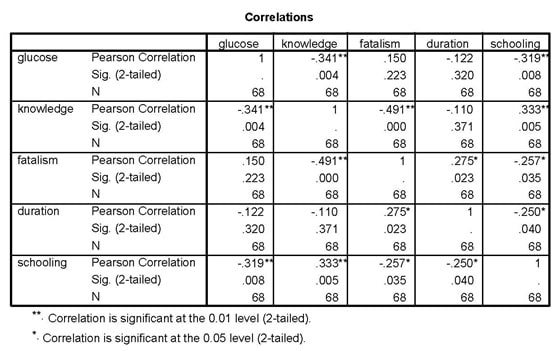### Regression 1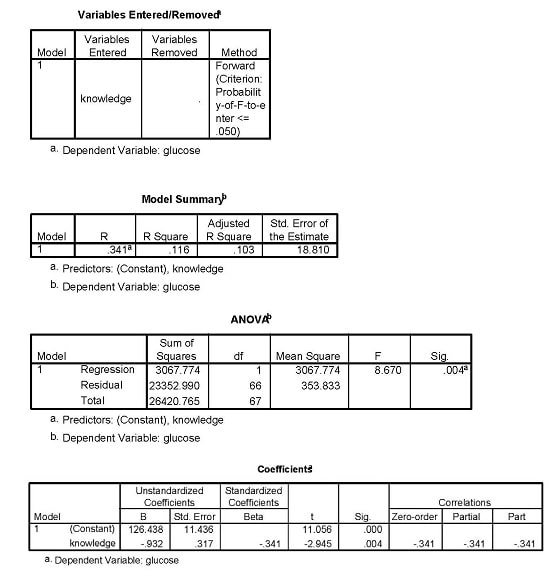### Regression 2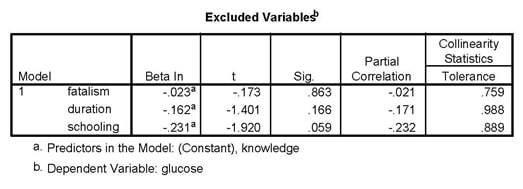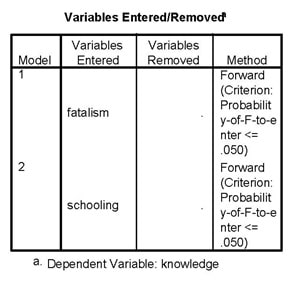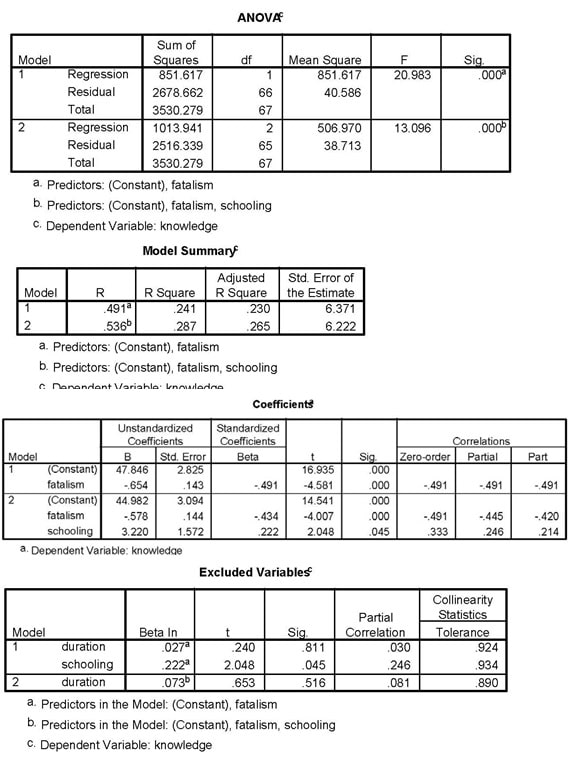### Regression 3